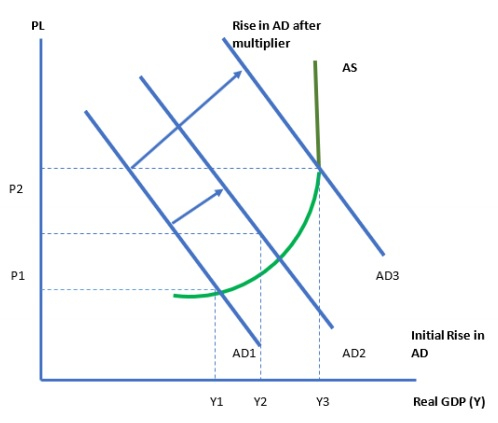# What is Keynesian Multiplier and how does it work?

According to the Keynesian Multiplier, a growth in variables is proportional of private consumption expenditure, investment expenditure, or net government spending raises the total Gross Domestic Product (GDP) by an amount greater than the increase in private consumption expenditure, investment expenditure, or net government spending. Consequently, if private consumption spending grows by 10 units, the overall gross domestic product (GDP) would increase by a factor of greater than 10.## Key Points Summary

• When the government spends more money, according to Keynesian philosophy, the economy will grow faster and more efficiently.

• As per the Keynesian multiplier theory, the net impact is higher than amount of dollar that the government has spent so far.

• Critics of this theory argue that it fails to take into consideration the fact that governments fund expenditures via taxes or debt problems.

## The Keynesian Multiplier is calculated in the following way −

Because the multiplier is dependent on both the marginal inclination to spend and the marginal propensity to save, the value of the multiplier varies.

## Savings Tendency on the Margin

The marginal propensity to save is the difference between total savings and total income as a consequence of a change in total income. In the case of an increase in an individual's income, the marginal propensity to save (MPS) is a measure of the percentage of increased income that the individual saves rather than spends on goods and services. MPS is computed using the formula MPS = ΔS/ΔY.

$$MPS=\frac{\Delta S}{\Delta Y}$$